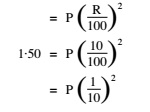# RRB ALP 2018 Practice Test Papers | Arithmetic Questions (Day-7)

Dear Aspirants, Here we have given the Important RRB ALP & Technicians Exam 2018 Practice Test Papers. Candidates those who are preparing for RRB ALP 2018 can practice these Arithmetic Questions to get more confidence to Crack RRB 2018 Examination.

[WpProQuiz 1506]

Click “Start Quiz” to attend these Questions and view Explanation

1. 3207 – 976 – 1781 =?

(A) 450

(B) 4012

(C) 442

(D) 2241

1. How many numbers are divisible by 7 between 4 and 100?

(A) 9

(B) 11

(C) 17

(D) 14

1. Two containers contain 60 and 165 litres of milk, respectively. Find the maximum capacity of a container which can measure the milk in each container an exact number of times.

(A)1 5

(B) 5

(C) 3

(D) 10

1. How much more is 2/5 of 105 than 3/4 of 48?

(A) 5

(B) 6

(C) 7

(D) 8

1. There are two grades A and B of workers in a workshop. Every worker contributes as many rupees as there are workers of his own category. If the total amount contributed is Rs. 196 including Rs. 16 contributed by the owner of the workshop, what is the total number of workers in that workshop?

(A) 18

(B) 14

(C) 12

(D) 10

1. There is 15 litres of a 20% alcohol mixture, 3 litres of water are added to it. What is the strength of the resultant volume?

(A) 17%

(B) 15%

(C) 18·5%

(D) 16·67%

1. If half the distance is to be covered in twice the time, what will be the ratio of the new speed to the original one?

(A) 1: 2

(B) 1: 4

(C) 2: 1

(D) 4: 1

1. The difference between simple interest and compound interest on a certain amount @ 10% p.a. for 2 years is Rs. 1·50. What is the amount?

(A) Rs. 750

(B) Rs. 150

(C) Rs. 1,500

(D) Rs. 7,500

1. The average of two numbers is 180. If one of them is half the other, the numbers are:

(A) 110, 220

(B) 120, 240

(C) 130, 260

(D) 140, 280

1. The breadth of a rectangle is 3/4 of its length. The area of the rectangle is 192 sq. metre. Its perimeter is:

(A) 16

(B) 12

(C) 56

(D) 742/3

Solution:

3207 – 976 – 1781=450

Numbers divisible by 7 between 4 and 100 are  7,14,21,28,35,42,49,56,63,70,77,84,91 and 98

The maximum capacity of a container which can measure the milk in each container an exact number of times, is the H.C.F. of 60 and 165.

H.C.F. of 60 and 165 = 15

2/5*105-3/4*48=6

The contribution by the workers in the workshop = 196 – 16 = Rs. 180

Let the number of workers in A grade be x and in B grade be y. ∴ x 2 + y2 = 180

Now, by putting x = 12 and y = 6, we get x 2 + y2 = 122 + 62 = 180 ∴ x + y = 12 + 6 = 18

The amount of alcohol in 15 litres of mixture = 15 × 20/100 = 3 litres

Volume of mixture on addition of 3 litres of water = 15 + 3 = 18 litres

Percentage of alcohol in new mixture = 3 × 100/18% = 16·67%

Let the distance = D and Time = T

∴ Original speed = D/T …(1)

As per question, New speed = (D /2) /(2 × T) = 1/4 * D/T

∴Reqd. Ratio = New speed: Original speed = 1/4* D/T : D/T = 1: 4

Let the principal sum be Rs. P. Then ∴ Difference between C.I. and S.I. for 2 years isP = 1·50 × 100 = Rs. 150

Let the number be x.

X+x/2=360

3/2 x=360

x=240, x/2=120

Let the length of rectangle = l metre,

∴l× 3/4 *l = 192 m2⇒ l2 = 4/3 × 192 = 256 = (16)2∴ l = 16 m

∴ Perimeter of rectangle = 2 (l + b) = 2 × (16 + 12) = 56 m

RRB ALP 2018 Practice Test Papers | Arithmetic Questions (Day-1)

RRB ALP 2018 Practice Test Papers | Arithmetic Questions (Day-2)

RRB ALP 2018 Practice Test Papers | Arithmetic Questions (Day-3)

RRB ALP 2018 Practice Test Papers | Arithmetic Questions (Day-4)

RRB ALP 2018 Practice Test Papers | Arithmetic Questions (Day-5)

RRB ALP 2018 Practice Test Papers | Arithmetic Questions (Day-6)